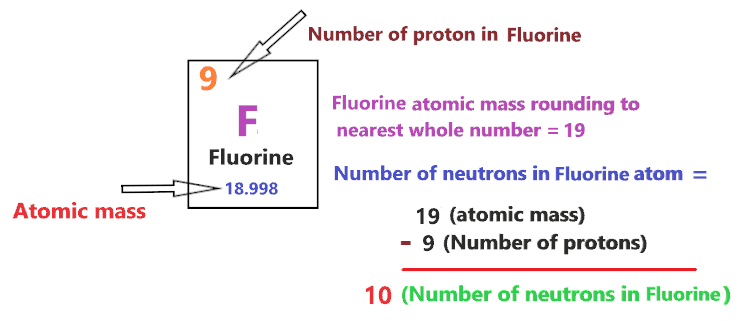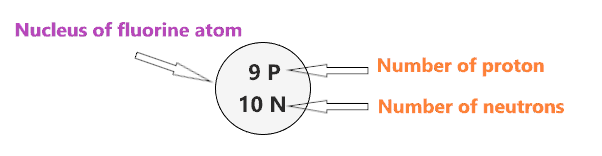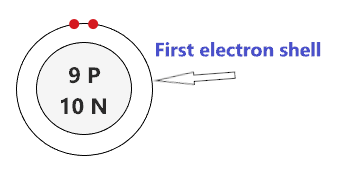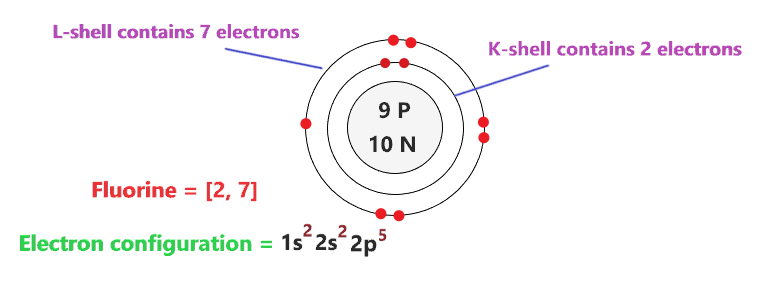# Bohr model of Fluorine atom - How to draw Fluorine(F) Bohr-Rutherford diagram?

Home  > Chemistry Article > Fluorine Bohr model

The Bohr Model of Fluorine(F) has a nucleus that contains 10 neutrons and 9 protons. This nucleus is surrounded by two-electron shells named K-shell and L-shell. The outermost shell in the Bohr diagram of Fluorine contains 7 electrons that also called valence electrons.

 Name Fluorine Bohr Model Number of neutrons 10 Number of protons 9 Number of electrons 9 Total electron shells 2 Electron in the First shell(K) 2 Electrons in the Second shell(L) 7 Total valence electrons in Fluorine 7
Page Contents

## How to draw Bohr Model of Fluorine(F)?

Bohr model describes the visual representation of orbiting electrons around the small nucleus. It used different electron shells such as K, L, M, N…so on. These shells hold a specific number of electrons, the electron shell which is closest to the nucleus has less energy and the electron shell which is farthest from the nucleus has more energy.

Bohr diagram is very interesting and easy to draw. Here, we will draw the Bohr diagram of the Fluorine atom with some simple steps.

## Steps to draw the Bohr Model of Fluorine atom

1. Find the number of protons, electrons, and neutrons in the Fluorine atom

Protons are the positively charged particles and neutrons are the uncharged particles, both these are constituents of the atom nuclei. Electrons are the negatively charged particles that orbit the nucleus of an atom

To find the number of protons an atom contains, just look at their atomic number.

If the atomic number of atom is 24, then proton will also be 24, if atomic number is 26, then proton will also be 26.

So, the atomic number for Fluorine is 9, hence, the number of protons in the Fluorine atom is also 9.Now, to determine the number of neutrons in an atom, use this formula.

⇒ Number of neutrons in atom = Atomic mass of the atom(rounded to the nearest whole number) – Number of proton in an atom

For example, An atom have 9.911 atomic mass and 5 protons.

Then, to find the number of neutron, round the atomic mass to the near whole number, so, atomic mass 9.911 round to 10.

= (10 – 5 protons) = 5 number of neutrons in the atom

Now, To get the number of neutrons in a Fluorine atom, look at its atomic mass which is 18.998 rounded to 19, and the number of protons in Fluorine is 9.

∴ Hence, the number of neutrons in Fluorine atom = (19 – 9) = 10It should be noted that “The number of electrons in a neutral atom is equal to the number of protons”.

So, the Fluorine atom is neutral, hence, its number of electrons will be equal to the number of protons which is Nine as we already discussed.

⇒ The number of electrons in Fluorine atom = 9

⇒ The number of protons in Fluorine atom = 9

⇒ The number of neutrons in Fluorine atom = 10

2. Draw the nucleus of an atom

A nucleus is a dense and small region that contains the number of protons and neutrons of an atom.

In this step, we have to draw a small circle that consists of a number of protons and the number of neutrons of a Fluorine atom.3. Draw the First electron shell

“An electron shell may be thought of as an orbit followed by electrons around an atom’s nucleus.”

The first electron shell is also called the K-shell, this is the closest shell to the nucleus of an atom and can hold a maximum of two electrons.

As we know, the Fluorine atom has a total of 9 electrons. So, put two electrons from it, in the first shell, next to each other.We have successfully drawn the first shell of the Fluorine atom that can hold 2 electrons. As Fluorine atoms have a total of 9 electrons, and from 9 electrons we have used two electrons in the first shell.

∴ (9 – 2) = 7 electrons

Therefore, we are left with 7 electrons, let’s put them in the next shell of the fluorine atom.

4. Draw the Second electron shell

The second shell also called the L-shell that can hold a maximum of 8 electrons. This shell is drawn after the first electron shell.

Since we already used two electrons in the first shell, now, we have to put the remaining 7 electrons in the second shell.

In the second electron shell, the electrons are added one at a time, starting from the top position then going in a clockwise direction.

In second shell, electrons are added one at a time in clockwise direction as a clock position – 12 o’clock, 3 o’clock, 6 o’clock, 9 o’clock positions.Once you place the electrons one at a time to each of the four sides(Top – Right – Bottom – Left], start pairing or doubling them.

So, we have to put 7 remaining electrons of the Fluorine atom in the second shell, hence, start from the top position, put electrons one at a time, and go in a clockwise direction(Top – Right – Bottom – Left). And, finally, start pairing.### Bohr model of Fluorine atom

That’s all, this is our Bohr model of the Fluorine atom that contains 9 protons and 10 neutrons in the nucleus region, and 9 electrons are orbited around the nucleus, two electrons in the first shell, and seven electrons in the second shell.

## Find Valence electron of Fluorine through its Bohr diagram

Valence electrons are found in the outermost shell of an atom and they can take participate in the formation of a chemical bond. These electrons have more energy compare to the inner shell electrons.

From the Bohr diagram of an atom, we can easily find the number of valence electrons in an atom by looking at its outermost shell.

So, we have to find a valence electron in the Fluorine atom, for this, look at its Bohr diagram.

Bohr’s diagram of Fluorine has only two electron shells (K and L), the inner shell is K-shell and the outermost shell is L-shell.

Hence, the electrons found in the L-shell of the Fluorine atom are its valence electrons because it is the outermost shell that also called the valence shell.

The L-shell or outer shell of the Fluorine Bohr model contains 7 electrons, therefore, the number of valence electrons in the Fluorine atom is 7.## Electron dot diagram of Fluorine atom

Electron dot diagram also called lewis structure which represents the valence electrons of atoms.

As, from the Bohr diagram of Fluorine, we got to know, it has 7 valence electrons.

So, just represent these 7 valence electrons around the Fluorine atom as a dot.## The electron configuration of Fluorine

Electron configuration is the distribution of electrons of an atom or molecule in atomic or molecule orbitals”

Fluorine has an atomic number of 9 and it contains a total number of 9 electrons. From the Bohr model of Fluorine, we know, 2 electrons are in the K-shell and 7 electrons are in the L-shell.

So, based on the shell, the electronic configuration of the Fluorine atom is [2, 7].

Or the electronic configuration of Fluorine is 1s22s22p5 since it contains a total of 9 electrons, the first two-electron will go in 1s orbital, the next two in 2s orbital, and the remaining five electrons will go in 2p orbital.

S orbital can hold maximum of 2 electrons and P orbital can hold maximum of 6 electrons.## FAQ

### How many electron shells a Fluorine Bohr model contains?

Electron shell also called energy level, you can find the number of electron shells for an element by knowing its period number in the periodic table.

The elements or atoms in the first period of the periodic table have one energy level or one electron shell, same as, the elements in the second period have two energy levels or two-electron shells and so on…

So, the Fluorine atom belongs to the 2nd Period in the periodic table, hence, the number of electron shells for the Bohr model of Fluorine will be 2 (K-shell and L-shell).

### What is the outer shell of the Bohr diagram of the Fluorine atom?

The outermost shell also called the valence shell, which contains valence electrons of the atom.

According to the Bohr diagram of Fluorine, the outer shell is L-shell which contains 7 valence electrons.

## Properties of Fluorine

1. At standard conditions, it appears as a pale yellow diatomic gas.
2. It is an extremely reactive and the most electronegative element in chemistry.
3. It has a cubic crystal structure.
4. Its electronegativity value at the Pauling scale is 3.98.
5. It is the lightest halogen and highly toxic in nature.

## Summary

• The Bohr model of Fluorine is drawn with only two electron shells, the first shell contains 2 electrons and the second shell contains 7 electrons.
• Fluorine is neutral and its atomic number is 9, hence, the number of protons and electrons available for its Bohr diagram is also 9.
• The number of neutrons for the Bohr diagram of Fluorine can be found by subtracting the number of protons from the atomic mass(rounded to the nearest whole).
• The electron configuration of Fluorine in terms of the shell is [2, 7], or in normal form, it is [He] 2s2 2p5.
##### Subscribe to Blog via Email

Join 2 other subscribers

Share it...